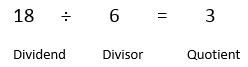# Different Terms for Add / Divide / Multiply / Subtract

There are a lot of different words used in maths which often confuse children who have not been told about them; most teachers will try and incorporate all of the following into their teaching but that doesn't help parents who have forgotten them or never got taught them!

Have a look at the following table of alternative words:

 + - X ÷ add take away multiply divide plus minus product share between altogether subtract times equal groups sum less than lots of total difference between more

Other words that are used include the following for division:There are others for the other operations but they will never turn up!

If you get a question like this, it is simply about finding the correct operation:

What is the product of seven and eight?

Product means multiply so we simply say 7 x 8 = 56.

Alternatively, look at this one:

What is the quotient when the total of 4 and 5 are shared equally by 3?

Be careful; if you provide children with this knowledge and especially if they don't speak perfect English, they can make errors. I've had children say 'it mentions the world 'total' so must be an addition sum' even though it clearly asks for you to work out what the total is and then carry out a separate operation. Under time pressure we all make mistakes so encourage your child to be careful and read questions all the way through.

The total of 4 and 5 is 9. Then share this equally by 3 and you get the quotient (the answer to a division sum) of 3.